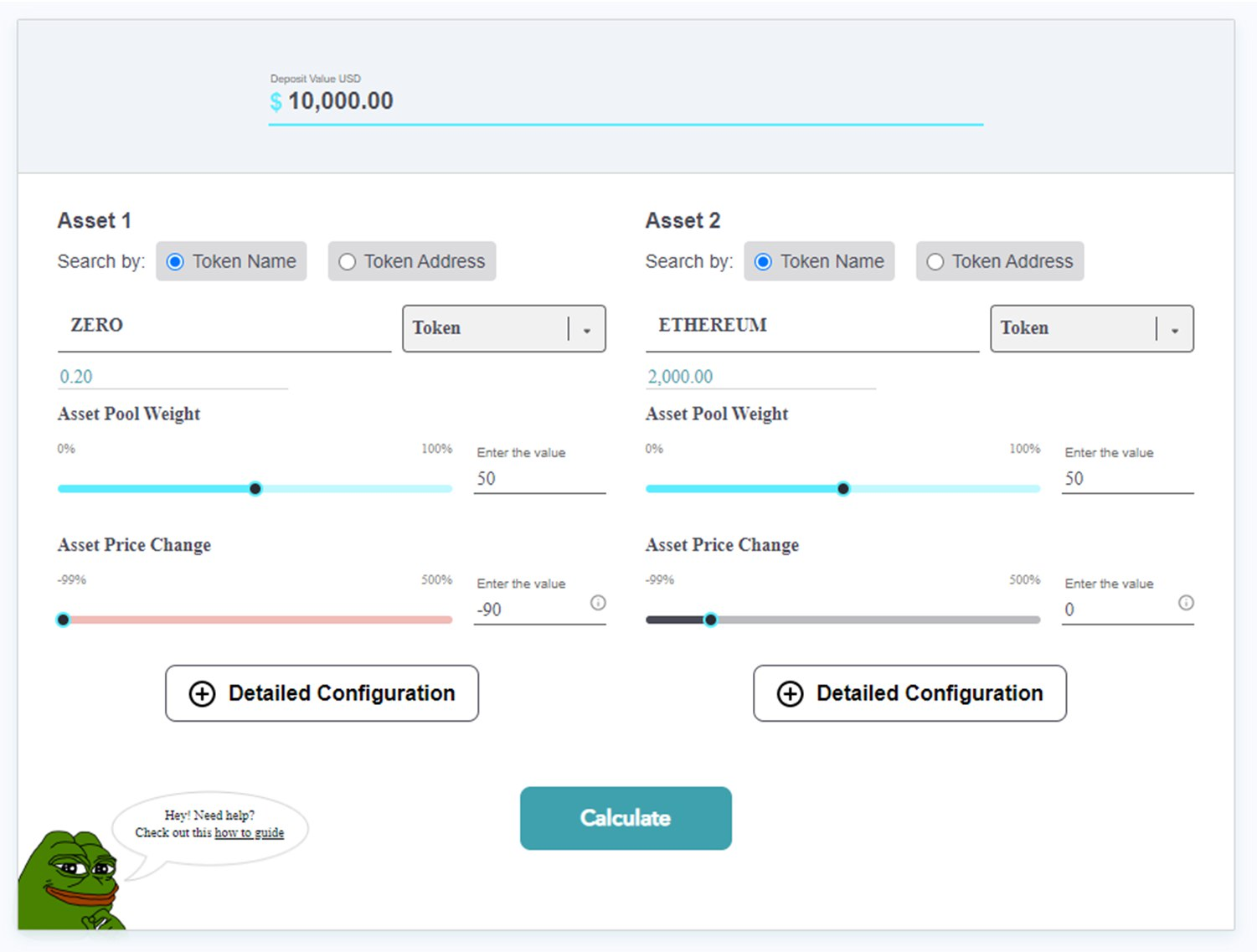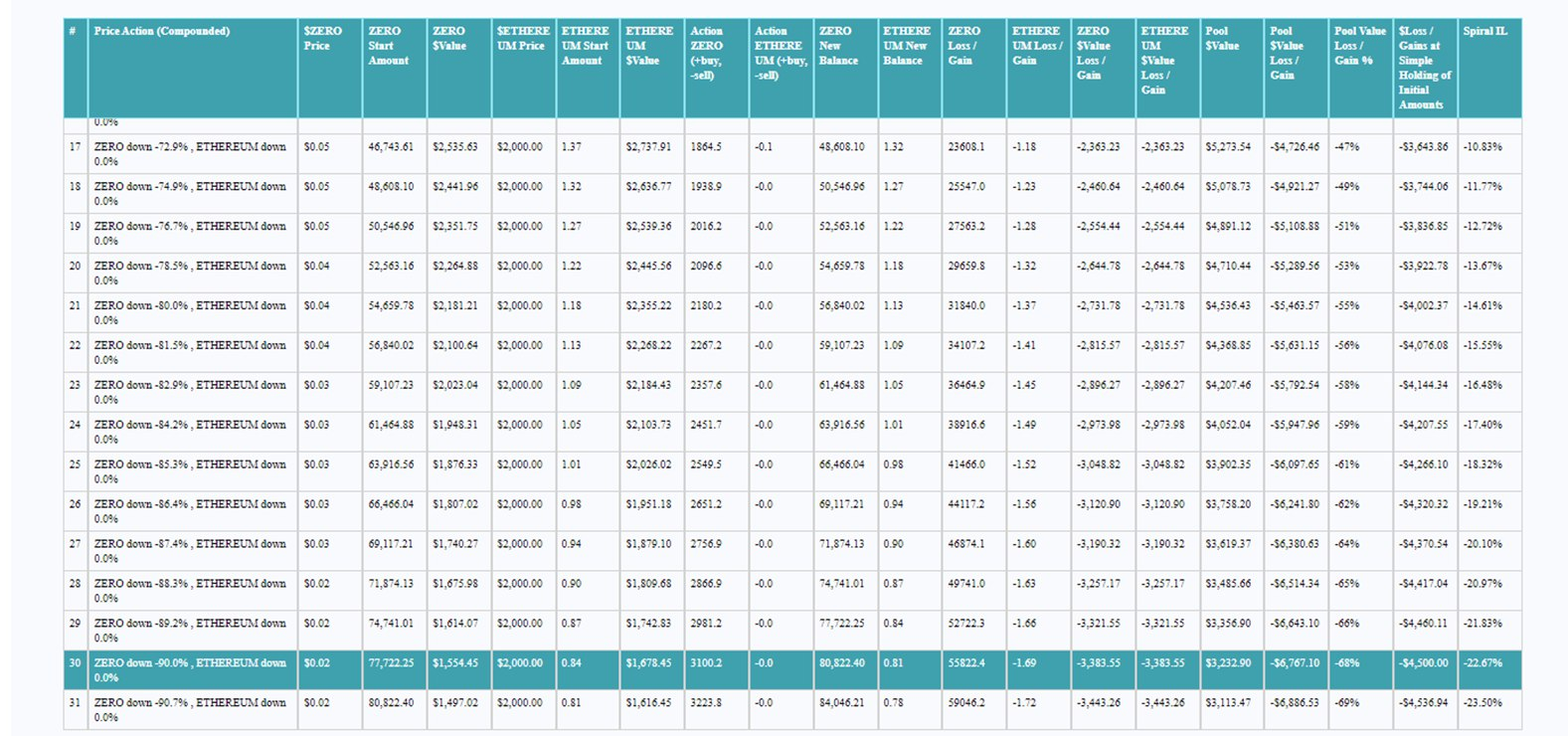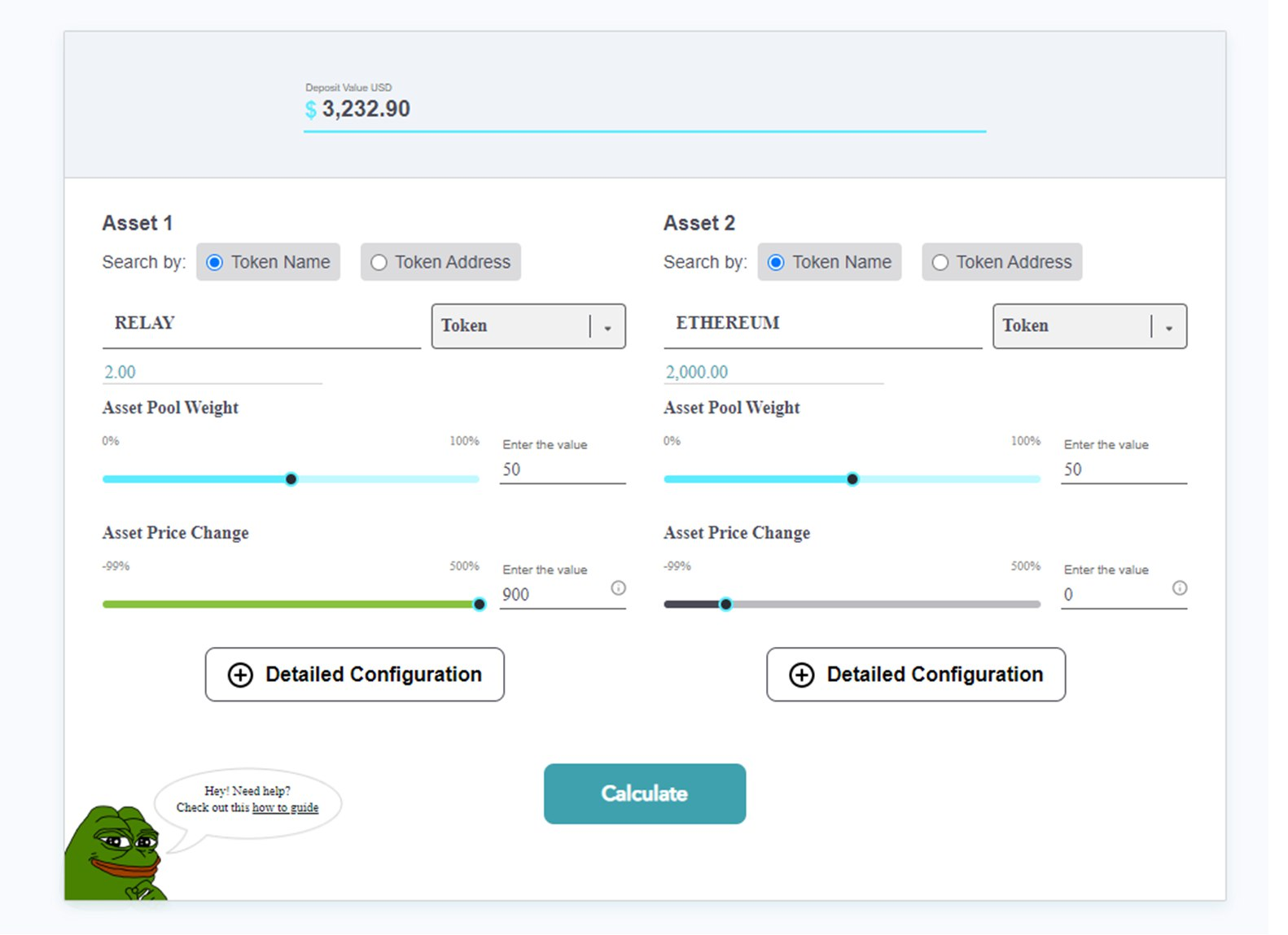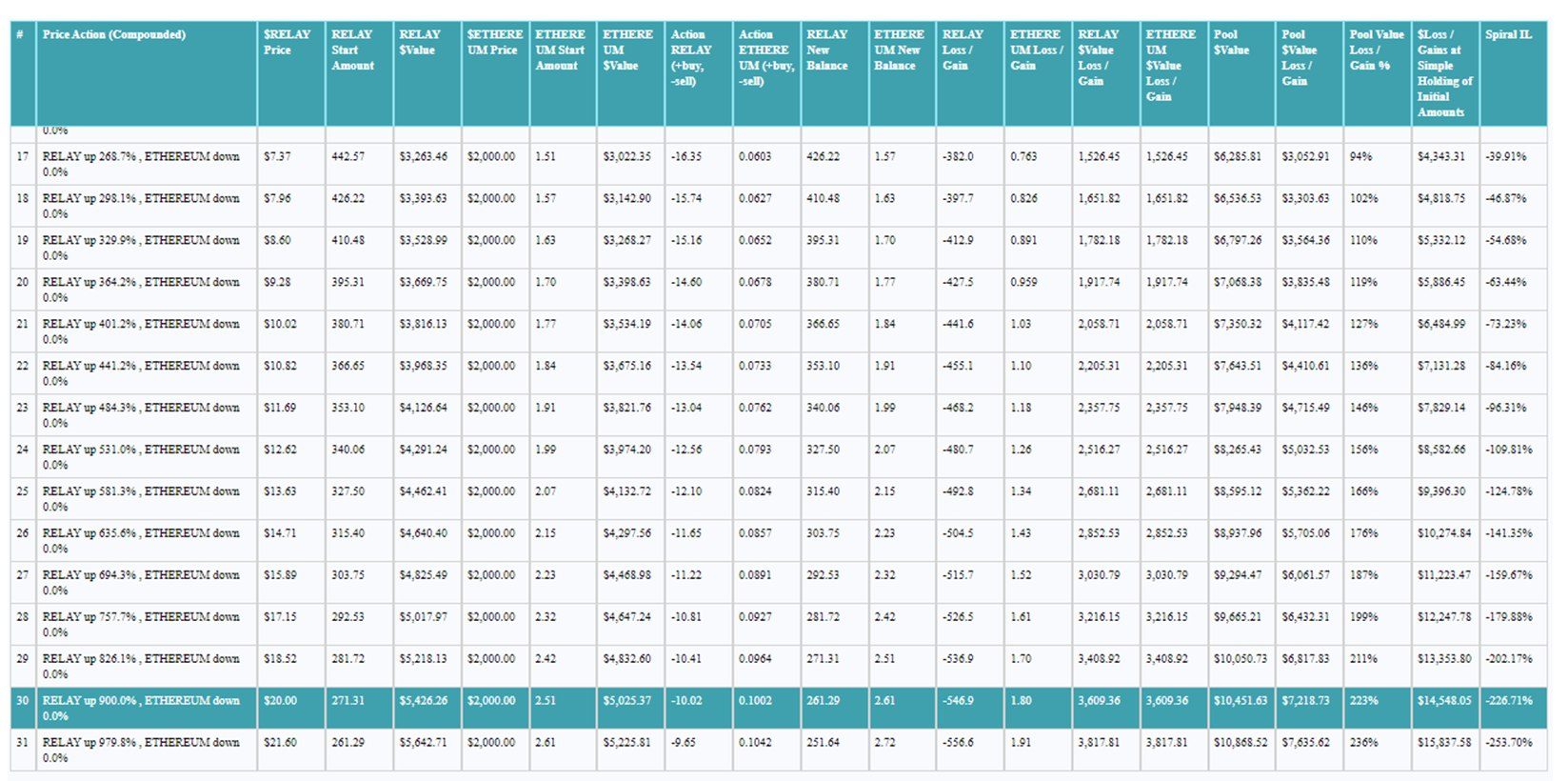## #Liquidity pools: Transitioning from ZERO to RELAY

### #Introduction

This page will explain the impact of impact of going from a ZERO to a RELAY liquidity pool ("LP" in the text) if you are currently in a ZERO pool, based on impermanent loss calculation ("IL" in the text) and dollar value of the assets composing the liquidity.

In summary: If you go out of the ZERO pool, exchange ZERO to RELAY quickly, and jump without waiting in a RELAY pool, you shouldn't notice any difference compared to the current situation of the ZERO pool (don't forget that all active ZERO pools will end). Then you are subjected on the RELAY price fluctuation (+ the paired token price action), same as today with ZERO.

### #Simulations

#### #ZERO-ETH pool

Figures/values of tokens are not real: Rounded and easy numbers were used (which are close to the real values) in order to make the explanation as simple as possible.Result of the different simulations with the ZERO token:If you had \$10,000 value LP and you entered with ZERO at 20c and ETH at \$2000, you would have started with 25,000 ZERO and 2.5 ETH (we’re not going to take rewards into consideration for this example). If the value of ZERO dropped 90% to 2c but ETH stayed at \$2000, then you would experience 22.67% IL. Your unrealized loss would be as follows:

• If you exited the LP with Zero at 2c and ETH at \$2,000 – then you would get 80,822.4 ZERO (a gain of 55,822.4 ZERO), and you would lose 1.69 ETH to leave a balance of 0.81 ETH
• The total USD value of your LP would be \$3,232.90 and you would have lost \$6,767.10 in USD value or 68%. So, if ZERO dropped 90% and ETH stayed stable at \$2,000, and you were in the LP, you would have a 68% USD value loss via IL when exiting which would leave you with an additional 55,822.4 ZERO which are now worth 90% less than when you entered, and 1.69 less ETH than what you entered with. The pool balanced by giving you more ZERO.

#### #Switching from the ZERO-ETH pool to the RELAY-ETH pool

RELAY is locked in value with ZERO at a ratio of 1:100, so for every 100 ZERO you get 1 RELAY token.

If you exited the LP at the time of the claim with the intent of switching to RELAY, here is what would happen:

• You would realize the IL and leave the LP with 80,822.4 ZERO and 0.81 ETH, you would then use your ZERO balance to claim RELAY at a ratio of 100:1, so for 80,822.4 ZERO you receive 808.224 RELAY tokens. At the time of the claim ZERO is worth 2c, and RELAY is worth \$2 (100X more). The 808.224 RELAY is worth \$1,616.448, as is 80,822.4 ZERO.

You can immediately enter the RELAY/ETH pool. The USD value of your tokens won’t have changed, so you enter the pool with a value of \$3,232.90 (equivalent to 80.8K ZERO and 0.81 ETH)

#### #Impact of the RELAY price action:

With the locking of value at 1:100 ratio in mind, for RELAY to recover its value to the same as when you entered the LP with ZERO at 20c, it would need to pump approx. 900% (pumping from 2c to 20c = 900%).A 900% pump on RELAY to get to \$20 (same as if ZERO went back 20c) and 0% pump on ETH = You lose some RELAY to IL, but you gain ETH. According to this chart, 826% pump actually gets back to the values of where you started, so at 826% you have a \$10,050 total pool value, a gain of 1.7ETH and a loss of 536.9 RELAY.

So it’s essentially the same as if you held on in the ZERO/ETH pool.
The IL doesn’t affect you, unless you stay out of the RELAY/ETH pool and just hold the tokens that you exited with.

Result of the different simulations with the RELAY token: